# While loops in Python

## This blog gives in-depth knowledge of how to while loops in Python. Read our blog to learn more...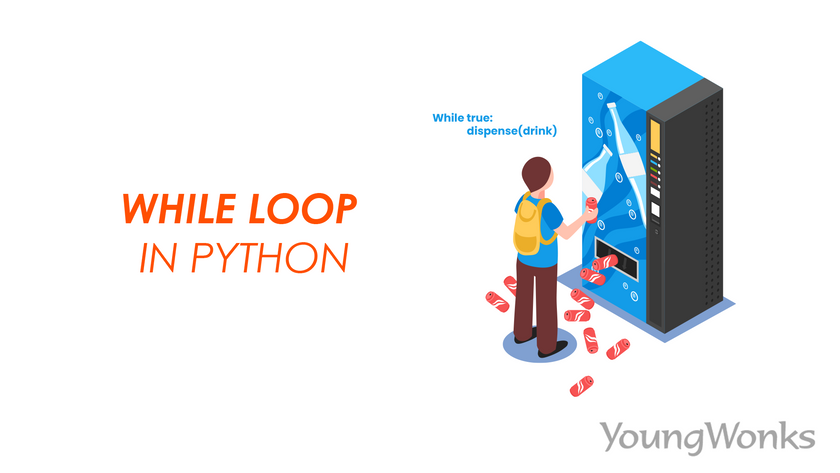Dec 05, 2022    By Team YoungWonks *

## What is a loop in programming?

A loop in programming runs a set of commands over and over again. The number of times a loop runs depends on the number defined by the user. There are two types of loops in programming i.e. for loops and while loops. These two loops are used in all the programming languages but differ in syntax. In this Python tutorial blog, we will discuss the use of while loop in Python programming.

## While loops in Python

The Python while loops are used to iterate a block of code until a specified condition is met. A condition can be either true or false. While loop is a conditional loop which runs until the condition in the loop is True. The condition written in a while statement is also known as boolean expression. Apart from integers, float and strings, boolean is one of the data type in Python which comprises of True and False values. This loop is used when it is difficult to predict how many times a block of code should be executed. This loop stops when the condition turns False.

Syntax:

while boolean expression:

body of the loop

In the above syntax we can observe that indentation is required for the block of statements which has to be repeated.

## Ways to use a while loop in Python

While loop can be used in two different ways i.e. while with a condition and a forever loop.

### 1. While loop with condition

Let us look into the flowchart and the following example to understand the logic.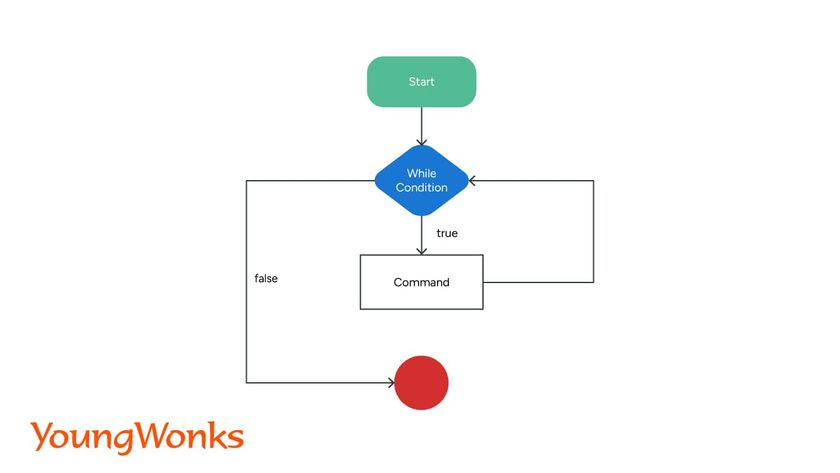In this the loop statements which is also known as the body of the loop is executed till the condition is True. The condition of the loop is evaluated repeatedly. The loop stops when the condition turns False.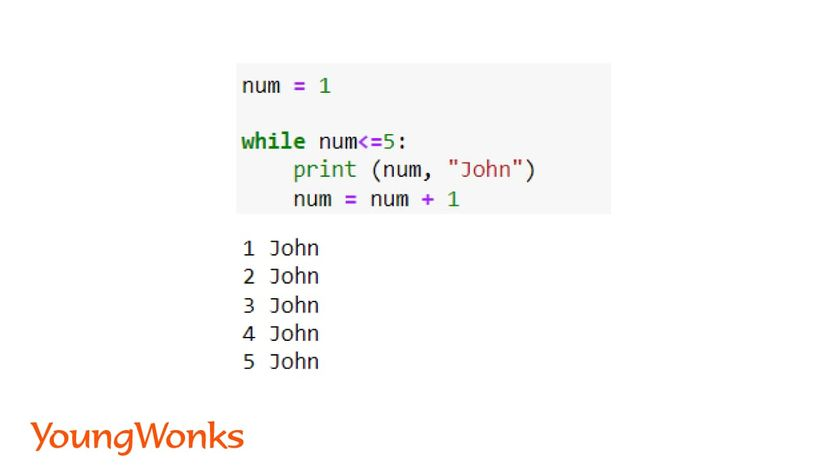In the above code the condition is true until the value of num is 5. Therefore, the code is repeatedly executed and it prints the numbers from 1 to 5.

### 2. Infinite Loop

This loop signifies that the condition is true forever. Therefore, the body of the loop keeps runs forever until it is stopped by the user.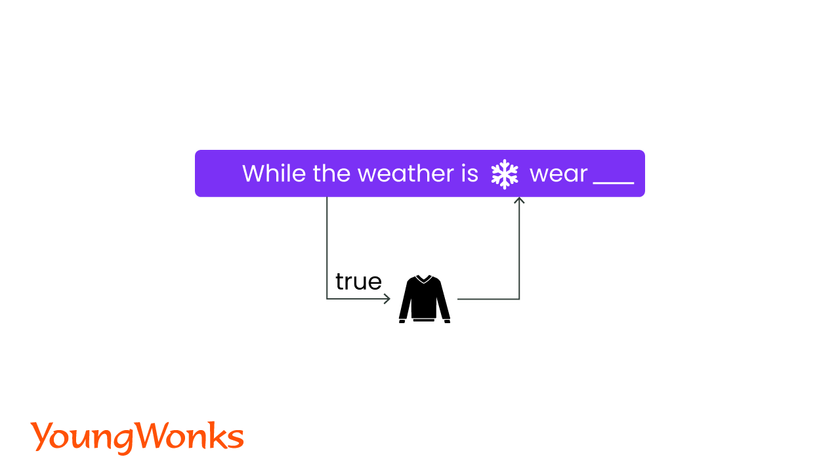Syntax:

while True:

body of the loop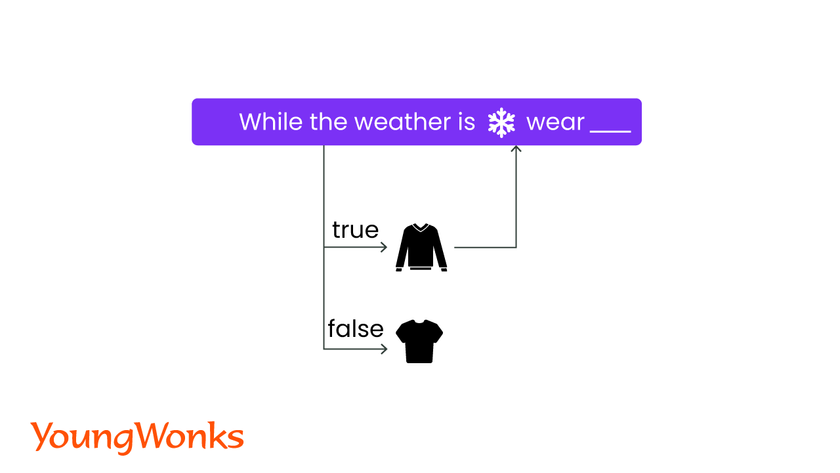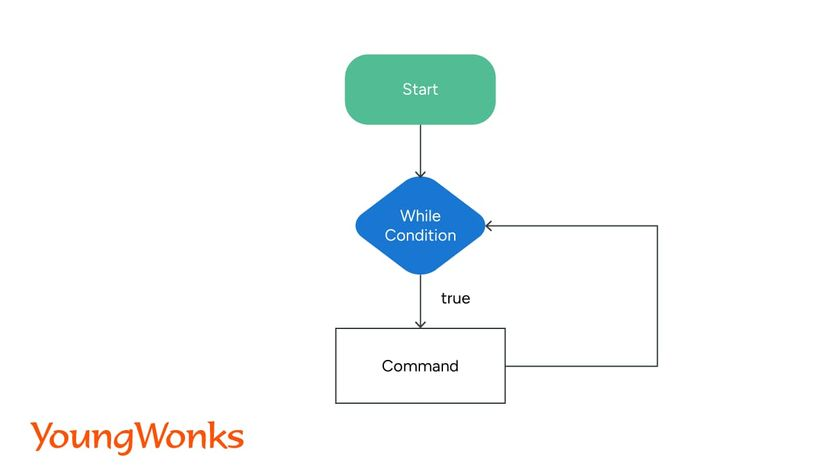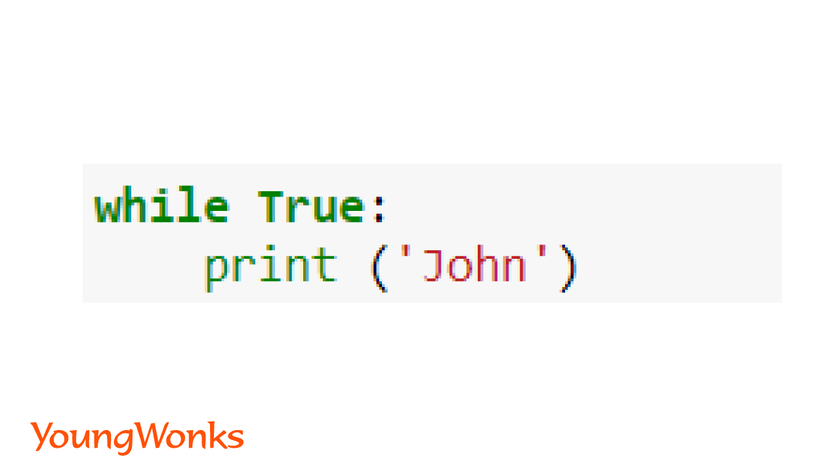### While loop using Python break keyword

We can also turn an infinite loop into a conditional loop in which we can stop the loop when a specific condition is met. If the condition is not met the loop keeps on running. We use the break keyword to stop a forever loop.

Syntax:

while True:

body of the loop

if boolean expression:

break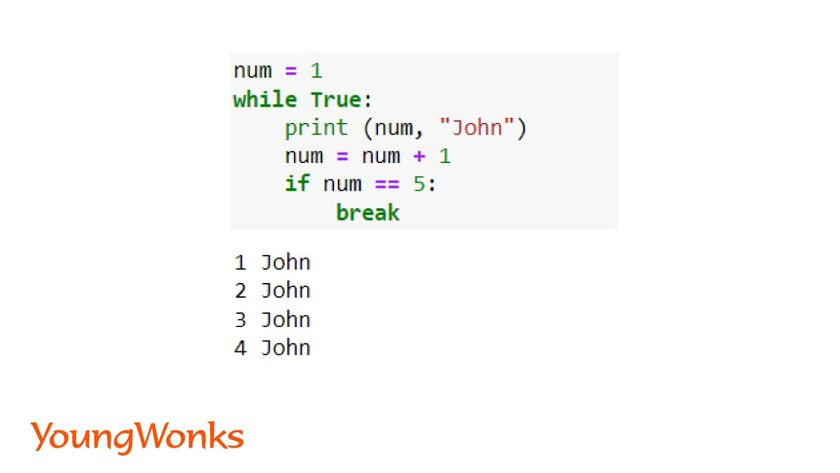In the above code, the Python break statement used here allows the loop to break when the value of num variable is 5.

Now let us look into the following example. We can notice that the value of num variable remains 1. The loop in this code is executed forever as the increment is missing from the body of the loop. Therefore, the condition never turns False. Such loops are also forever loops.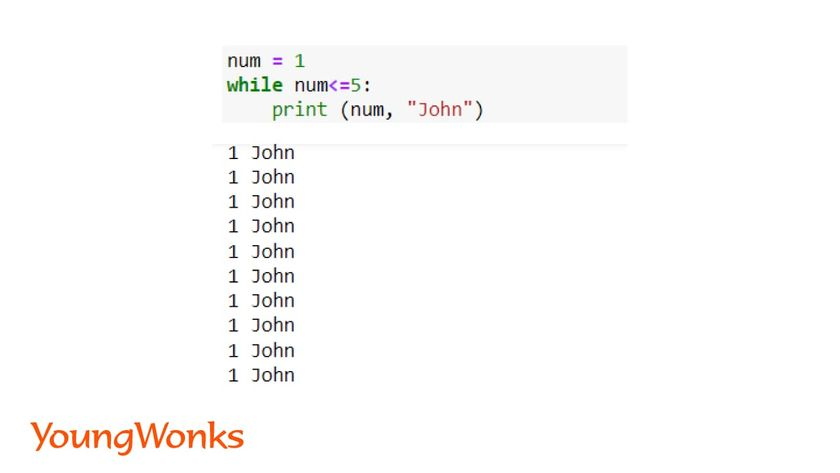## Other ways of using a while loop in Python

### 1. While loop with else clause

Else block can be used after the while loop. After the while loop is executed the code in the else clause is executed. It is not necessary if there is an output of the while loop, else statement will run irrespective.

Syntax:

while boolean expression:

body of the loop

else:

body of the condition

Let us look into the count down timer example given below. In the first example, the while loop and else clause is executed one after the other. In the second example though the while loop is False, the else clause is executed.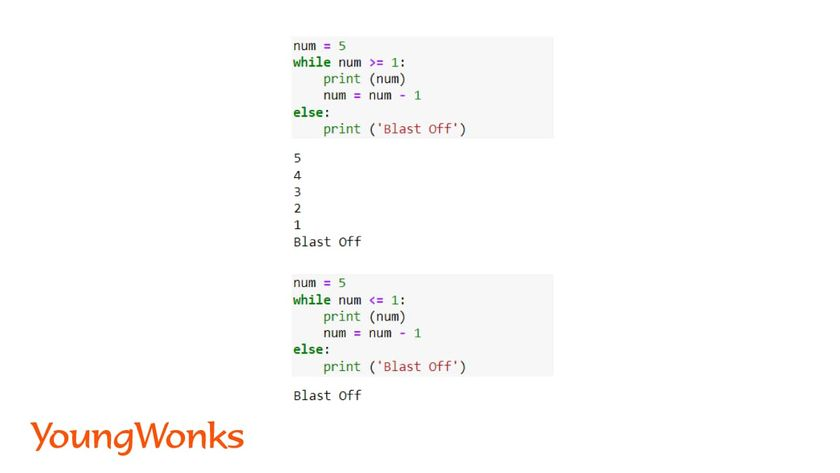### 2. While loop with continue statements

Continue statements are used in both the loops. It is used to end the current iteration in a loop and continue with the next iteration.

In the following example, number 0 is not printed as a condition is added to skip the iteration when the variables value is 0.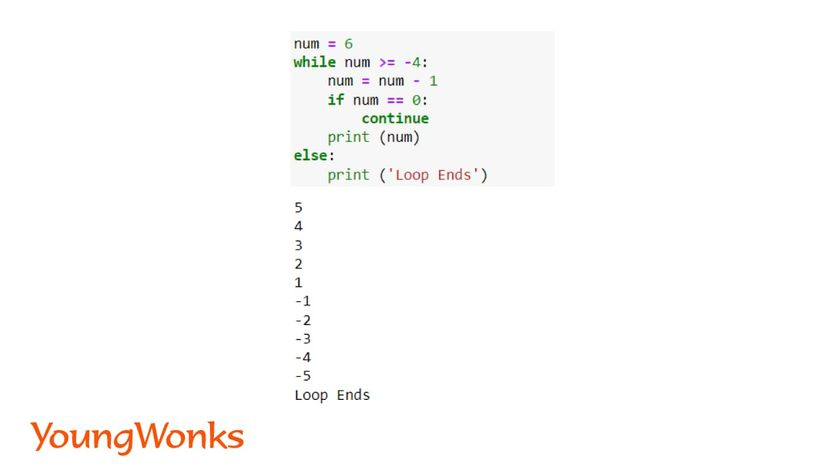## Summary

In this beginner Python blog on while loops we have discussed in detail about while loops and its syntax. Now you can apply this other applications such as Python functions, game development, data science and machine learning, etc. In the next blog we will discuss about Python for loops.

*Contributors: Written by Aayushi Jayaswal; Lead image by Shivendra Singh

This blog is presented to you by YoungWonks. The leading coding program for kids and teens.

YoungWonks offers instructor led one-on-one online classes and in-person classes with 4:1 student teacher ratio.

Sign up for a free trial class by filling out the form below:

By clicking the "Submit" button above, you agree to the privacy policy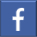Share on Facebook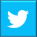Share on Twitter
help